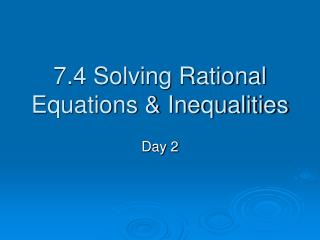# 7.4 Solving Rational Equations & Inequalities - PowerPoint PPT PresentationDownload Presentation7.4 Solving Rational Equations & Inequalities

7.4 Solving Rational Equations & InequalitiesDownload Presentation## 7.4 Solving Rational Equations & Inequalities

- - - - - - - - - - - - - - - - - - - - - - - - - - - E N D - - - - - - - - - - - - - - - - - - - - - - - - - - -
##### Presentation Transcript

1. Rational Inequalities • Solve for x using the calculator Set the equation equal to zero Graph in your calculator Where is the graph GREATER THAN zero? Use the trace function. You can also go to the table and look at the values to see when the y-values start to get larger than zero.

2. Rational Inequalities • Solve for x using the calculator Set the equation equal to zero Graph in your calculator Zero: x = 1/2 VA: x = 2 Step 2: Put the left hand side into y1 and the right hand side into y2. Step 3: Use the zero feature to find where the graph of y1 lies above the x-axis. Solution:

3. Rational Inequalities • Solve for x using the calculator Set the equation equal to zero Graph in your calculator Zero: x = -1.5 VA: x = -5 Step 2: Put the left hand side into y1 and the right hand side into y2. Step 3: Use the zero feature to find where the graph of y1 lies above the x-axis. Solution:

4. Rational Inequalities • Solve for x using the calculator Set the equation equal to zero Graph in your calculator Zero: x = 0 VA: x = 1 Step 2: Put the left hand side into y1 and the right hand side into y2. Step 3: Use the zero feature to find where the graph of y1 lies above the x-axis. Solution:

5. Rational Inequalities • Solve for x using the calculator Set the equation equal to zero Graph in your calculator VA: x = 7 VA: x = -5 Step 2: Put the left hand side into y1 and the right hand side into y2. Step 3: Use the zero feature to find where the graph of y1 lies above the x-axis. Solution:

6. Rational Inequalities Solve for x using the calculator From 1990 to 2001, the number d (in thousands) of doctors in the United States can be modeled by the function where t is the number of years since 1990. When were there fewer than 800,000 doctors? Step 1: Set up the inequality Step 2: Put the left hand side into y1 and the right hand side into y2. Step 3: Use the intersect feature to find where the graph of y1 lies below the graph of y2. Solution: In the years 1990-1999, there were fewer than 800,000 doctors

7. Rate – of – Work Problems It takes 7 hours for John to paint a house. Jerry can paint the same house in 5 hours. How long will it take them to paint the house if they work together? TIME or t = time it takes John and Jerry to paint the house together. Identify the variable Write an equation. Solve the equation.

8. In Class ASSIGNMENT • Homework: Page 600 #1 – 17 odds Worksheet – Homework if you don’t finish it in class